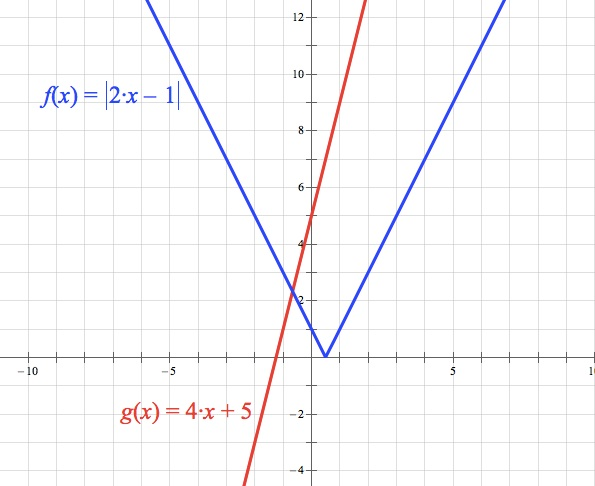Subject Test question:

|2x-1| = 4x+5 has how many numbers in its solution set?

A) 0
B) 1
C) 2
D) an infinite number
E) none of above

How do you find the answer algebraically without graphing it?

First of all, graphing this (and many questions on the Subject Tests) is a really good way to go. If you don’t have a graphing calculator of your own but you know how to use one (maybe your school has them?), talk to your teacher and see if you can borrow one for the test. The graphing solution, since I just can’t help myself, looks like this:Since the slope of the red line is steeper than the slope of the blue line, there will only be one intersection.

To solve this algebraically, you have to solve two equations:

2x – 1 = 4x + 5
–6 = 2x
3 = x

–(2x – 1) = 4x + 5
–2x + 1 = 4x + 5
–4 = 6x
–2/3 = x

Then you have to plug both values back into the original equation to ensure neither is extraneous. Hint: one will be!

|2(–3) – 1| = 4(–3) + 5
|–6 – 1| = –12 + 5
7 = –7

Oops! That’s not true! –3 is an extraneous solution.

|2(–2/3) – 1| = 4(–2/3) + 5
|–4/3 – 1| = –8/3 + 5
7/3 = 7/3

Yep—that one worked just fine.